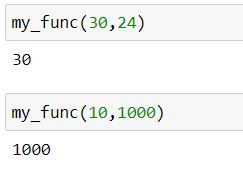top of page
Search
• Ekta Aggarwal

# Lambda functions in Python

Lambda functions or lambda expressions are sophisticated one liner functions which can help you by avoiding writing UDFs.

Syntax:

lambda input values : resultant expression

Topics covered:

### Understanding with examples!

Task: Create a function to calculate the cube of a number.

Usual way without lambda functions -

We have created a function named cube which returns the cube of a number.

```def cube(x):
return x**3

cube(3)```

Using lambda expression:

In the following code lambda is a keyword, x : indicates that x is our input value;

x**3 is the resultant output by lambda expression.

We have stored this lambda expression in cube_func .

`cube_func = lambda x: x**3`

To calculate cube of a number we have called our cube_func and passed 3 to get its cube.

On calling cube_func, we are assigning x as 3 and our result would be 3**3 i.e. 27.

`cube_func(3)`### It is not always necessary to store the lambda expression

In the following code we have written a lambda expression to get cube of a number and have called it directly by specifying (3 ) outside it.

`(lambda x: x**3) (3)`But this is only for one-time use. If we need to re-use this lambda expression later then we MUST store it.

### Lambda function having multiple arguments

A lambda expression can take multiple input values.

In the following code our lambda expression takes 2 input values and assigns them to x and y and returns x*y as the result.

```my_func = lambda x,y : x*y

my_func(3,2)```### Lambda function with list comprehension

We can also provide an if-else list comprehension inside a lambda function.

Task: Create a lambda expression which returns maximum of the 2 numbers:

In the following code we are using list comprehension: which is as follows:

value_if_true condition_to_be_evaluated else value_if_condition_is_fal

`my_func = lambda x,y : x if x > y else y`

Above code compares two elements x and y and provides the maximum element final output!

```my_func(30,24)
my_func(10,1000)```Lambda functions are mainly used with 3 Python functions: map, reduce and filter.

### map function:

Map( ) in Python is used to apply an in-built or user defined or lambda functions to an iterator object (eg. lists, sets, tuples etc.). It is highly useful to write shorter and elegant Python codes avoiding the need of using for loops.

Task: Return the square of the numbers in a list.

We can pass a lambda function inside our map( ) function:

```numbers =  [3,4,5,6]
result = map(lambda x: x**2, numbers)
print(list(result)) ```

Output:

`[9, 16, 25, 36]`

Let us create 2 lists:

```list1 = [1,2,3,4]
list2 = [10,20,30,40]```

If we are passing 2 iterators then lambda function must be able to take 2 variables as input (in our case x and y) and following lambda function returns product of the corresponding elements:

`list(map(lambda x,y: x*y,list1, list2))`

Output:

`[10, 40, 90, 160]`

### Reduce function

To find cumulative sum or products or to compare elements in a list sequentially, reduce( ) function in Python can be your saviour!

It belongs to library functools so we need to import it first.

`from functools import reduce`

Syntax:

reduce (function , list, initial_value <optional>)

Let us create our list:

`mylist = [10,20,30,40]`

Let us apply lambda function in reduce:

`reduce(lambda x,y:x+y,mylist)`

How is it working?

Lambda function firstly sums first two elements of our list: 10 and 20 and stores the resultant output i.e. '30' in memory.

Next, it adds the next element i.e. 30 to our stored output, thus it stores the output 60 in memory.

Finally it takes the fourth element from the list i.e. 40 and adds it to 60, so 100 is our output!### filter function

filter( ) in Python is used to apply an in-built or user defined or lambda functions to an iterator object (eg. lists, sets, tuples etc.) to filter the iterator object.

Syntax:

filter( function, iterator object)

where first argument i.e. function needs to return True or False, or zero or non-zero values which will be used to filter our iterator object. Let us create our list named salary:

`salary = [4000,2565,2345,7653,1000,825]`

Task: Filter only those salaries which are more than 4000.

In the following code we have used list comprehension:

Value_when_condition_is_true IF condition_to_be_evaluated ELSE value_when_condition_is_false

`list(filter(lambda x: False if x< 4000 else True, salary))`

Above lambda function returns the value False when salary is less than 4000, otherwise it will return True.

Output:

`[4000,7653]`